Thermogravimetry and the Negative Temperature Dependence of Gravity | OMICS International
Journal of Physical Mathematics

# Thermogravimetry and the Negative Temperature Dependence of Gravity

Dmitriev AL*

Department of Mechanics and Optics, St. Petersburg National Research University of Information Technologies, Russia

*Corresponding Author:
Dmitriev AL
Department of Mechanics and Optics
St. Petersburg National Research University of Information Technologies
Russia
Tel: 812-3154071
E-mail:
[email protected]

Received Date: October 12, 2015; Accepted Date: October 28, 2015; Published Date: October 31, 2015

Citation: Dmitriev AL (2015) Thermogravimetry and the Negative Temperature Dependence of Gravity. J Phys Math 6:148. doi:10.4172/2090-0902.1000148

Copyright: © 2015 Dmitriev AL. This is an open-access article distributed under the terms of the Creative Commons Attribution License, which permits unrestricted use, distribution, and reproduction in any medium, provided the original author and source are credited.

Visit for more related articles at Journal of Physical Mathematics

#### Introduction

It is shown that data of thermo gravimetric measurements confirm the negative temperature dependence of gravity. The accounting of this dependence is necessary for increase of accuracy of the thermo gravimetric analysis.

The thermogravimetric analysis based on exact weighing of the heated sample is widely applied in researches of physical and chemical properties of materials [1-3]. The first stage of thermo gravimetric measurements is receiving a basic curve – temperature dependence of weight of the empty holder of a sample, for example, of a crucible. At data processing of temperature measurements the basic curve is subtracted from experimental temperature dependence of weight. Out of areas of change of phase structure of substance in which there are sharp changes of its weight, the main reason for the monotonous increasing temperature dependence of weight is considered action of forces of buoyancy. Meanwhile, physical temperature dependence of weight of bodies [4-6] has essential impact on measurements of weight and has to be taken into account in the exact thermogravimetric analysis; this circumstance was noted by Grumazescu . At creation of a basic curve the seeming mass M of the holder (crucible) in air is equal M=m -ρV, where m-the mass of a crucible, V-its volume and ρ-air density. Temperature change of the seeming weight

The thermogravimetric analysis based on exact weighing of the heated sample is widely applied in researches of physical and chemical properties of materials [1-3]. The first stage of thermo gravimetric measurements is receiving a basic curve – temperature dependence of weight of the empty holder of a sample, for example, of a crucible. At data processing of temperature measurements the basic curve is subtracted from experimental temperature dependence of weight. Out of areas of change of phase structure of substance in which there are sharp changes of its weight, the main reason for the monotonous increasing temperature dependence of weight is considered action of forces of buoyancy. Meanwhile, physical temperature dependence of weight of bodies [4-6] has essential impact on measurements of weight and has to be taken into account in the exact thermogravimetric analysis; this circumstance was noted by Grumazescu . At creation of a basic curve the seeming mass M of the holder (crucible) in air is equal M=m -ρV, where m-the mass of a crucible, V-its volume and ρ-air density. Temperature change of the seeming weight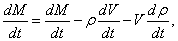(1)

Where V=V0(1+βt), β-volume expansion coefficient of material of a crucible. Temperature dependence of ρ(t) is represented by known expression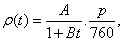(2)

Where A=0.0012932 g/cm3, B = 0.00367 K-1, p - air pressure in mm Hg . By normal ( p=760 ) air pressure Eq. 1 may be represent as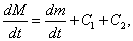Where C1= -V0βA(1+βt)-1, and C2= V0(1+βt)AB

For numerical estimates we will use results of work  according to which the seeming change of mass of a porcelain crucible with the weight m=4 g and volume 1.5 cm3 measured on Shevenar's thermo scales in the temperatures range 200-1000°C is equal 4.0.10-6 gK-1. Volume coefficient of expansion of porcelain β=9.10-6 K-1  and, for example, at t=200°C, the values of coefficients C1.2 are equal C1= -1.0.10-8 gK-1 and C2= 7.1.10-6 gK-

As the absolute values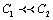, the main contribution to the seeming change of mass of a crucible is made by the effects of buoyancy described by coefficient C2. Obviously, the consent of the experimental and provided settlement data is possible only at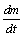= -3.1.10-6 gK-1.This fact directly confirms established in [4-6] negative temperature dependence of weight of bodies. Temperature change of weight of the holder, it is generally connected with change of temperature of a porcelain crucible, the assessment of size of relative temperature change of the weight of porcelain from where follows the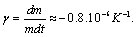Sign and an order of the specified size correspond to data of measurements of physical temperature dependence of weight of various metals [4-6], and also PZT . For more exact quantitative estimates of influence of temperature dependence of weight on results of thermogravimetric measurements, the accounting of the sizes, forms, masses, physical and thermodynamic characteristics as holder, and the studied sample is necessary [8,9]. Temperature dependence of physical weight of bodies will allow to establish the reasons of anomalies of thermogravimetric dependences with bigger degree of reliability and to increase the accuracy of the gravimetric analysis.

#### References

Select your language of interest to view the total content in your interested language

### Article Usage

• Total views: 12733
• [From(publication date):
November-2015 - Jan 20, 2020]
• Breakdown by view type
• HTML page views : 8744Can't read the image? click here to refresh